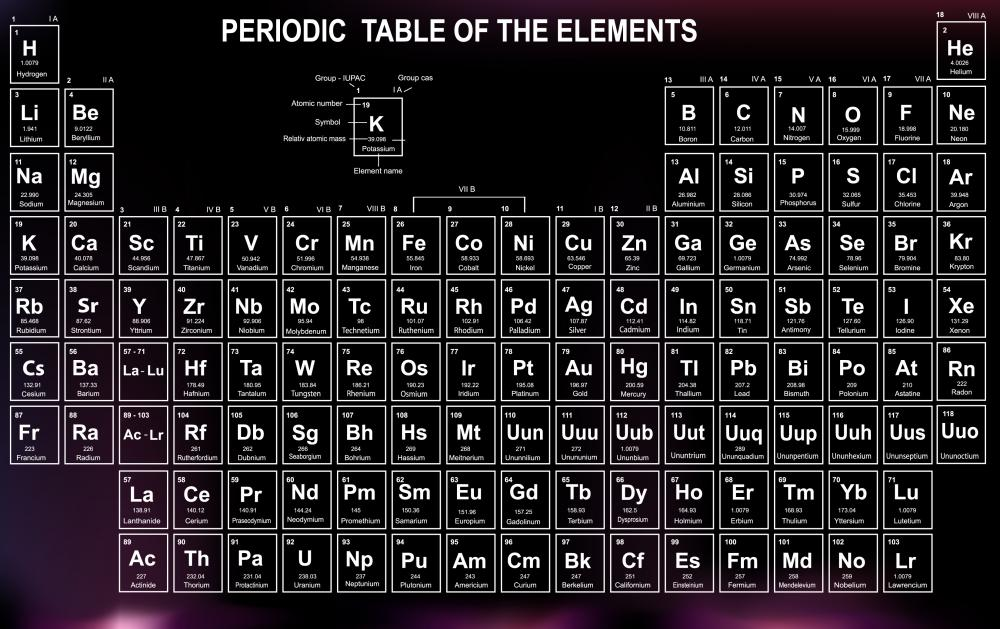Brendan McGuigan

Avogadro's number, also known as Avogadro's constant, is defined as the quantity of atoms in precisely 12 grams of 12C. The designation is a recognition of Amedeo Avogadro, who was the first to state that a gas' volume is proportional to how many atoms it has. This number is given as 6.02214179 x 1023 mol-1.Amedeo Avogadro hypothesized that even very different elements have the same number of molecules in the same volume of an ideal gas.

Amadeo Avogadro lived in the early 19th century and was an Italian savant known for his role in many different scientific disciplines. His most famous statement is known as Avogadro's Law, and is a hypothesis that states, "Equal volumes of ideal or perfect gasses, at the same temperature and pressure, contain the same number of particles, or molecules."

This is an intriguing hypothesis, because it says that quite different elements, such as nitrogen and hydrogen, still have the same number of molecules in the same volume of an ideal gas. While in real world settings this is not strictly true, it is statistically quite close, and so the ideal model still has a great deal of value.

The constant can be expressed as (p1)(V1)/(T1)(n1) = (p2)(V2)/(T2)(n2) = constant; where p is the pressure the gas is at, T is the temperature it is at, V is the volume of gas, and n is the number of molar units.

Part of Avogadro's genius, and while this number was named after him, is that he was able to see this fundamental relationship long before the experimental evidence was available to validate it. His innate understanding of the nature of ideal gasses was astounding, and it wasn't until decades later that experimental evidence finally supported his hypothesis.

In the 1860s, more than 50 years after Avogadro first made his hypothesis, the Austrian high school teacher Josef Loschmidt calculated how many molecules were in a single cubic centimeter of a gas under typical pressure and temperature. He determined this to be approximately 2.6X1019 molecules, a number now known as Loschmidt's Constant, and which has since been expanded to 2.68677725X1025 m-3.

Throughout the early years of the 20th century, a search was undertaken to discover the precise value of Avogadro's number. Molecules were still largely theoretical entities to many scientists until the early part of the 20th century, and so actually determining the value through experiment was not feasible. Once it became feasible, however, it was immediately apparent that the value was important, as it reflected on the fundamental nature of ideal gasses.

The name "Avogadro's number" was first used in a paper from 1909, by the scientist Jean Baptiste Jean Perrin, who later went on to win the Nobel Prize in Physics in 1926. He stated in the paper that, "The invariable number N is a universal constant, which may be appropriately designated 'Avogadro's Constant.'"

For years leading up to the 1960s, there was some dispute as to the actual value of this number. Some factions used oxygen-16 to base their calculation on, while others used a naturally occurring isotope of oxygen, leading to slightly different values. In 1960, the constant was changed to be based on carbon-12, making the number much more regular.

## You might also Likeanon354052

How does the avogardo number vary with temperature and pressure?

anon122934

How can you go from molecule/square nanometer to micromol/square meter? I ask this because in the HPLC for Pharmaceutical Scientists the Authors Prof. Kazakevich and Prof. LoBrutto give a wrong coefficient!

Amphibious54

@ Istria- Avogadro's number, or Avogadro's mole, is essential to understanding stoichiometry. You make a very good point about the importance of the number being based on the mole mass of 12C. The mole scale makes it possible to deal with the infinitesimally small atomic mass units (AMU) of a substance in a more digestible manner. We rarely deal with chemicals or substances in such small quantities that they can be measured in AMUs.

I would also like to add that you can use the mole scale to work with and convert molecules or ionic compounds of substances. For example, if you wanted to determine the number of N2 molecules in 10.50 grams of N2 gas, you could use Avogadro's conversion scale (the answer is approximately 2.26*10^23 molecules by the way). You would simply divide the number of grams of N2 (10.50) by the number of Grams per mole of N2 (28.00), then multiply the whole number by Avogadro's constant.

istria

@ Anon30180- Avogadro's number is a universally accepted chemistry constant that is most commonly used as a conversion factor for determining moles in Chemistry. One mole of a substance is 6.022X10^23 pieces (atoms, molecules, ionic compounds, even specs of dust for that matter).

The reason that the constant is based on carbon-12 is because there are exactly 12.0000 grams of carbon-12 per mole of carbon-12 (6.022 X 1023 carbon-12 molecules= 12 grams). This is the only isotope in the periodic table that has this property of a whole number in grams per mole of atoms. This makes the conversion the most accurate, and it allows people to use the atomic mass number of an atom as the number of grams per mole of that element, i.e., the mole mass of the element gold (Au) is 196.967 g/mol.

anon30180

So, why do some folks still use the oxygen based value for Avadagro's constant, 6.023 x 10^23??